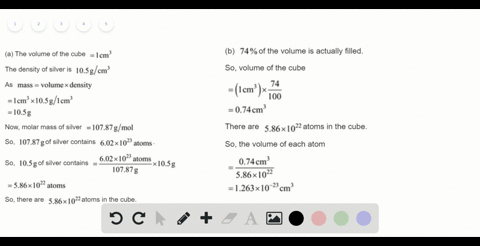Sign up for our free STEM online summer camps starting June 1st!View Summer Courses### (a) You are given a cube of silver metal that mea…

02:18Auburn University Main Campus

Need more help? Fill out this quick form to get professional live tutoring.

Get live tutoring
Problem 108

Consider a sample of calcium carbonate in the form of a cube measuring 2.005 in. on each edge. If the sample has a density of 2.71 $\mathrm{g} / \mathrm{cm}^{3}$ , how many oxygen atoms does it contain?

$6.457 \times 10^{24}$ atoms

## Discussion

You must be signed in to discuss.

## Video Transcript

So in this problem, we are given with a sample off calcium cargo on it in the form of a cube which miss er to plant all five inch on each ends. And the sample has a density ofthe thiss. I have to find out how many oxygen atoms does the Semple content. So what we have to do first is since you're given, uh, age measurement of the cube, which is the form of the calcium carbonate. First you have to go find out the volume of the calcium carbonate from this Mr Hman and from there using the density, we confined the mass of calcium carbonate. From there we can find a number of malls of caution. Garment. From there we can find the number of Mel's off oxygen. And from there we can find the number of Aussie Ian Adams. So first to first, which is will find a following of calcium carbonate. So the volume first you'LL have to find it in inch Q which is? The volume will be the old cube of this. It opened all five inch, um and kill which will give us available six point Cyril six zero inch cubes now we have to convert this just centimeter Q. Because our density is given in Gramp er, Centimeter Cube. So the volume of calcium carbonate in cm Q will be six point zero six zero Time. Six months here, six zero in cube time. So one inch Cubas, sixteen point three eight seven centimeter cubes, one inch cubes to sixteen hundred seven centimeter cubes. So he has multiplied thiss by these two. Get ah Avello off one thirty two point oik centimeter Q. So this is the volume ofthe calcium carbonate in Sentiment Cube. Now we have to find a massive calcium carbonate, which will be the volume times density. So the volume is one thirty two point. Is there a way to see him killed? Time's a density, which is two point seven one Gramp er, same cube. It will be equal to three. Fifty seven point nine for Graham. So this is the mass off two. Fifty seven point nine. For Graham, this is the massive calcium government. From here we can find a number of malls of calcium carbonate. So the number of mall of Kill Shankar when it will be the mass of calcium carbonate three point two fifty seven bond. Nine for Graham over the molar mass of calcium carbonate, which is one hundred point two one. And graham are mall, and it will give us a bell off three point five seven six more. So this is the number of more of Hailsham Covenant. Now, from here, we can find the number of mall off oxygen in this. So here you can see that one molecule of collision comet contains three atoms off oxygen. That means one more of calcium current will will contain three mole off oxygen atom. And this much Matloff Gulshan camera will contain these times three matloff oxygen. So the number of matloff oxygen atom will be three point five seven six more times tree, which is equal to ten point seven to eight more. So this is the number of Mall of Oxygen. Now, from here, we can find the molecule of the number of atom of oxygen. Says you know that in one mole off in a substance we have six months, zero times ten to the twenty three at home. That means this much more off oxygen. We will have temper seventy eight more times six point four times ten to the power twenty three Adam Fire One more So to give us the hell off. Six point four six. Time to tend it. About twenty four Adam off oxygen. So this is the number ofthe atom off oxygen in the symbol of cash uncovered given.